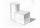# Diagonal + surface area - math problems

#### Number of problems found: 43

• Space diagonalThe space diagonal of a cube is 129.91 mm. Find the lateral area, surface area and the volume of the cube.
• Body diagonalFind the cube surface if its body diagonal has a size of 6 cm.
• Body diagonalCalculate the volume and surface of the cube if the body diagonal measures 10 dm.
• Body diagonalThe cuboid has a volume of 32 cm3. Its side surface area is double as one of the square bases. What is the length of the body diagonal?
• Wall diagonalCalculate the length of wall diagonal of the cube whose surface is 384 cm square.
• Sphere areaA cube with edge 1 m long is circumscribed sphere (vertices of the cube lies on the surface of a sphere). Determine the surface area of the sphere.
• Three faces of a cuboidThe diagonal of three faces of a cuboid are 13,√281 and 20 units. Then the total surface area of the cuboid is.
• Axial sectionAxial section of the cylinder has a diagonal 40 cm. The size of the shell and the base surface are in the ratio 3:2. Calculate the volume and surface area of this cylinder.
• Body diagonal - cubeCalculate the surface and cube volume with body diagonal 15 cm long.
• Cube wallThe perimeter of one cube wall is 120 meters. Calculate the surface area and the body diagonal of this cube.
• Cube and sphereCube with the surface area 150 cm2 is described sphere. What is sphere surface?
• Cube diagonalsDetermine the volume and surface area of the cube if you know the length of the body diagonal u = 216 cm.
• Cuboid diagonalCalculate the volume and surface area of the cuboid ABCDEFGH, which sides a, b, c has dimensions in the ratio of 9:3:8. If you know that the diagonal wall AC is 86 cm, and the angle between AC and space diagonal AG is 25 degrees.Calculate the volume and surface area of a regular quadrangular prism 35 cm high and the base diagonal of 22 cm.
• Surface area of cylinderDetermine the lateral surface of the rotary cylinder which is circumscribed cube with edge length 5 cm.
• Nice prismCalculate the cuboid's surface if the sum of its edges is a + b + c = 19 cm and the body diagonal size u = 13 cm.
• Surface area of the topA cylinder is three times as high as it is wide. The length of the cylinder’s diagonal is 20 cm. Find the surface area of the top of the cylinder.
• Four sided prismCalculate the volume and surface area of a regular quadrangular prism whose height is 28.6cm and the body diagonal forms a 50-degree angle with the base plane.
• Cube wallCalculate the cube's diagonal if you know that one wall's surface is equal to 36 centimeters square. Please also calculate its volume.
• Cuboid diagonalsThe cuboid has dimensions of 15, 20 and 40 cm. Calculate its volume and surface, the length of the body diagonal and the lengths of all three wall diagonals.

Do you have an interesting mathematical word problem that you can't solve it? Submit a math problem, and we can try to solve it.

We will send a solution to your e-mail address. Solved examples are also published here. Please enter the e-mail correctly and check whether you don't have a full mailbox.

Please do not submit problems from current active competitions such as Mathematical Olympiad, correspondence seminars etc...